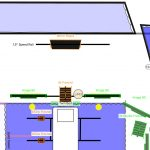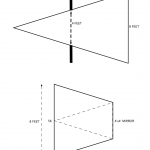# Lighting

Posted on by
Back to Lighting...

## on stage use of a mirror (4 replies and 1 comment)

Rod 2 months ago

Hello Folks

for my upcoming shot. I am trying to lessen the fall off of my main light by using a 4x4 mirror. (emulating Mr. Deakins work in the computer room in Skyfall). my only concern is will the light cover a 4x6 window? the distance between the window and the mirror would be approx 7 feet (see pic)? thanks .

Rod 2 months ago

here is the picdmullenasc 2 months ago

The amount of spread depends on how close the light is to the mirror. I think you'll be fine -- think of it this way, if you stand at each end of the 6' window looking from the inside out at the mirror and you can see the 5K fresnel reflected in it, then the light will hit that end of the window.

There is probably some math that would tell the angle of spread... Or maybe you can figure out the spread if the 5K was pointed directly at the window -- at a distance from the 5K to the mirror plus the distance of the mirror to the window -- and then imagine a 4'x4' cutout cookie placed at the halfway point.

dmullenasc 2 months ago

Using some math, you can split the beam in half to draw a right triangle, and so if there is a 4'x4' cookie in the way of the beam at the halfway point of 7' (if you imagine no bouncing into a mirror, just the 5K being twice as far away at 14'), that's two right triangles, each 7' x 2' on the adjacent and opposite sides, which works out to a 15.945 degree angle... so when the beam continues out to 14', the other side of the right triangle is 4', so the beam diameter is 8' at 14' away.  So it will fill a 6' wide window.

dmullenasc 2 months ago

Math isn't my strong point but this roughly demonstrates what I'm talking about.  This assumes of course that the full beam diameter is larger than the 4'x4' hole it passes through at 7' away, which it is -- the Mole-Richardson catalog says that a 5K fresnel at 15' away has a beam diameter of 13.7' at full flood, and in this case, it just has to fill a 6' wide area at 14' away. So the 4'x4' mirror is cutting that down to a 8'x8' square.

You can see why if you radically increase the distance of the lamp to the mirror but shorten the distance of the mirror to the window, the spread will be much narrower. And vice-versa. But what makes your situation easy to calculate is that the light is above the window so you have an equal distance from the light to the mirror and from the mirror to the window.

But I could be miscalculating all of this...